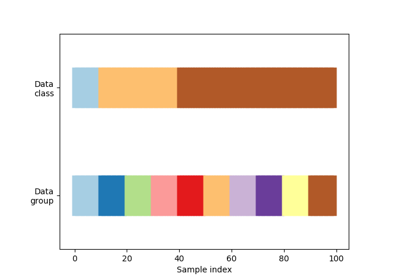# sklearn.model_selection.GroupKFold¶

class sklearn.model_selection.GroupKFold(n_splits='warn')[source]

K-fold iterator variant with non-overlapping groups.

The same group will not appear in two different folds (the number of distinct groups has to be at least equal to the number of folds).

The folds are approximately balanced in the sense that the number of distinct groups is approximately the same in each fold.

Parameters: n_splits : int, default=3 Number of folds. Must be at least 2. Changed in version 0.20: n_splits default value will change from 3 to 5 in v0.22.

LeaveOneGroupOut
For splitting the data according to explicit domain-specific stratification of the dataset.

Examples

>>> from sklearn.model_selection import GroupKFold
>>> X = np.array([[1, 2], [3, 4], [5, 6], [7, 8]])
>>> y = np.array([1, 2, 3, 4])
>>> groups = np.array([0, 0, 2, 2])
>>> group_kfold = GroupKFold(n_splits=2)
>>> group_kfold.get_n_splits(X, y, groups)
2
>>> print(group_kfold)
GroupKFold(n_splits=2)
>>> for train_index, test_index in group_kfold.split(X, y, groups):
...     print("TRAIN:", train_index, "TEST:", test_index)
...     X_train, X_test = X[train_index], X[test_index]
...     y_train, y_test = y[train_index], y[test_index]
...     print(X_train, X_test, y_train, y_test)
...
TRAIN: [0 1] TEST: [2 3]
[[1 2]
[3 4]] [[5 6]
[7 8]] [1 2] [3 4]
TRAIN: [2 3] TEST: [0 1]
[[5 6]
[7 8]] [[1 2]
[3 4]] [3 4] [1 2]


Methods

 get_n_splits([X, y, groups]) Returns the number of splitting iterations in the cross-validator split(X[, y, groups]) Generate indices to split data into training and test set.
__init__(n_splits='warn')[source]
get_n_splits(X=None, y=None, groups=None)[source]

Returns the number of splitting iterations in the cross-validator

Parameters: X : object Always ignored, exists for compatibility. y : object Always ignored, exists for compatibility. groups : object Always ignored, exists for compatibility. n_splits : int Returns the number of splitting iterations in the cross-validator.
split(X, y=None, groups=None)[source]

Generate indices to split data into training and test set.

Parameters: X : array-like, shape (n_samples, n_features) Training data, where n_samples is the number of samples and n_features is the number of features. y : array-like, shape (n_samples,), optional The target variable for supervised learning problems. groups : array-like, with shape (n_samples,) Group labels for the samples used while splitting the dataset into train/test set. train : ndarray The training set indices for that split. test : ndarray The testing set indices for that split.

## Examples using sklearn.model_selection.GroupKFold¶Visualizing cross-validation behavior in scikit-learn